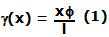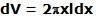## Saturday, May 2, 2009

### Irodov Problem 1.312Since one end is fixed all points on that end will be at the same place after the distortion. Consider a line OA on the fixed side and a corresponding parallel line O'B on the free end. After the distortion, B moves to a location B' - this induces a shear strain of magnitude BB'/l. Another point X on AB moves to location X' and so this area experiences a shear XX'/l. Thus, the cylinder experiences different shear strains at different distances from the axis.

Suppose that the point X is at a distance x from the axis of the cylinder. The length of XX' is approximatelyand so the shear strain experienced at point X is given by,The energy per unit volume due to this shear strain is given by. Consider an infinitesimally thin cylindrical section of thickness dx at point X. The volume of this infinitesimally thin cylindrical section is. The total energy can be computed by integrating the energy contained in all such infinitesimally thin sections and hence this given by,